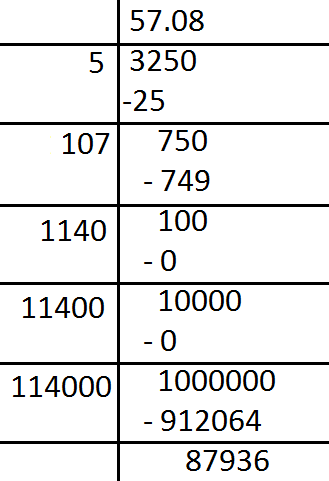Find the square root of $3250$by division method.Verified
147.9k+ views
Hint: In mathematics a square root of a number is a number$y$ such that ${y^2} = x$, in other words a number $y$whose square is $x$. The term whose square root is being considered is known as the radicand. The radicand underneath the radical sign every$+ ve$ number$x$has two square roots $\sqrt x$which is $+ ve$ and $- \sqrt x$ which is $- ve$. Together, these two roots are denoted as $\pm \sqrt x$. Although the principal of square root of a $+ ve$ number is only one of its two square roots designation. The square root for $+ ve$ $x$ the principal square root can also be written in exponent notation as ${\left( x \right)^{\dfrac{1}{2}}}$.
The square root of $- ve$ number can be discussed within the framework of complex numbers more generally, square roots can be considered in any context in which notation of squaring of some mathematical objects is defined. The $x$ indicates algebras of matrices endomorphism rings among other mathematical equations.Note: The square root of a $+ ve$ number is usually defined as the side length of a square with area equal to the given number. But the square shape is not necessary for it. If one of two similar planar inclined objects have the area times greater than another then the ratio of their linear sizes is $\sqrt a$.